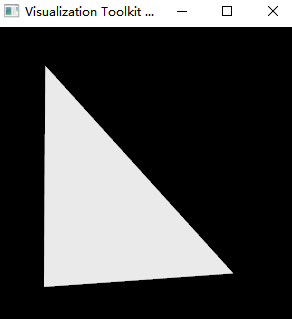# Is it possible to set thickness for vtkPolygon

I want to use `vtkPolygon` in my project, and the code is pasted as following:

``````import vtkmodules.all as vtk

polyData = vtk.vtkPolyData()

vps = vtk.vtkPoints()
polygon = vtk.vtkPolygon()

polygon.GetPointIds().SetNumberOfIds(3)

vps.InsertNextPoint(0, 0, 0)
polygon.GetPointIds().SetId(0, 0)
vps.InsertNextPoint(1, 0, 0)
polygon.GetPointIds().SetId(1, 1)
vps.InsertNextPoint(0, 1, 0)
polygon.GetPointIds().SetId(2, 2)
polygons = vtk.vtkCellArray()
polygons.InsertNextCell(polygon)
polyData.SetPoints(vps)
polyData.SetPolys(polygons)

mapper = vtk.vtkPolyDataMapper()
mapper.SetInputData(polyData)
actor = vtk.vtkActor()
actor.SetMapper(mapper)
render = vtk.vtkRenderer()
renWin = vtk.vtkRenderWindow()
iren = vtk.vtkRenderWindowInteractor()
iren.SetInteractorStyle(vtk.vtkInteractorStyleTrackballCamera())
iren.SetRenderWindow(renWin)
iren.Initialize()
iren.Start()
``````

I can correctly show this polygon:However, when I rotate this polygon, if the normal of this polygon is parallel to the view up direction of vtkCamera, the polygon will disappear. Like:I wonder whether it is possible to set a thickness to the vtkPolygon. So that the polygon will look like a line when the normal of polygon is parallel to the view up direction of vtkCamera.

In a word, I don’t want this polygon disappear.

Any suggestion is appreciated~~

You can perform a modeling operation like vtkLinearExtrusionFilter to transform the 2D polygon into a 3D wedge. Extrude in the direction of the polygon normal.